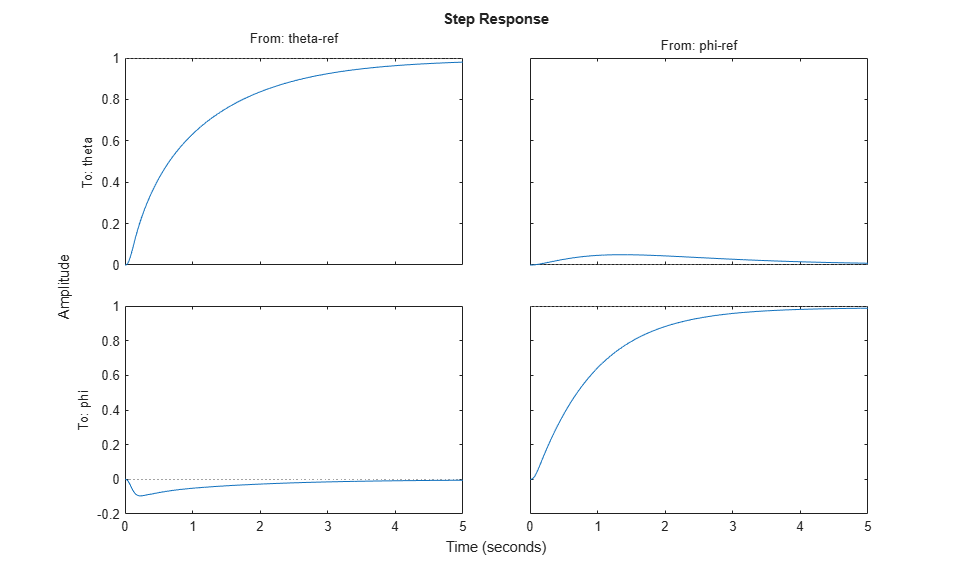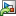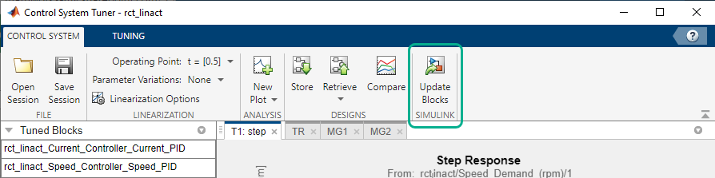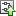## Validate Tuned Control System

When you tune a control system using `systune` or Control System Tuner, you must validate the results of tuning. The tuning results provide numeric and graphical indications of how well your tuning goals are satisfied. (See Interpret Numeric Tuning Results and Visualize Tuning Goals.) Often, you want to examine other system responses using the tuned controller parameters. If you are tuning a Simulink® model, you must also validate the tuned controller against the full nonlinear system. At the command line and in Control System Tuner, there are several tools to help you validate the tuned control system.

### Extract and Plot System Responses

In addition to the system responses corresponding to your tuning goals (see Visualize Tuning Goals), you can evaluate the tuned system performance by plotting other system responses. For instance, evaluate reference tracking or overshoot performance by plotting the step response of transfer function from the reference input to the controlled output. Or, evaluate stability margins by examining an open-loop transfer function. You can extract any transfer function you need for analysis from the tuned model of your control system.

#### Extract System Responses at the Command Line

The tuning tools include analysis functions that let you extract responses from your tuned control system.

For generalized state-space (`genss`) models, use:

For an `slTuner` interface, use:

In either case, the extracted responses are represented by state-space (`ss`) models. You can analyze these models using commands such as `step`, `bode`, `sigma`, or `margin`.

For instance, suppose that you are tuning the control system of the example Multiloop Control of a Helicopter. You have created an `slTuner` interface `ST0` for the Simulink model. You have also specified tuning goals `TrackReq`, `MarginReq1`, `MarginReq2`, and `PoleReq`. You tune the control system using `systune`.

```AllReqs = [TrackReq,MarginReq1,MarginReq2,PoleReq]; ST1 = systune(ST0,AllReqs); ```
```Final: Soft = 1.12, Hard = -Inf, Iterations = 73 ```

Suppose also that `ST0` has analysis points that include signals named `theta-ref`, `theta`, `phi-ref`, and `phi`. Use `getIOTransfer` to extract the tuned transfer functions from `theta-ref` and `phi-ref` to `theta` and `phi`.

```T1 = getIOTransfer(ST1,{'theta-ref','phi-ref'},{'theta','phi'}); step(T1,5) ```The step plot shows that the extracted transfer function is the 2-input, 2-output response from the specified reference inputs to the specified outputs.

For an example that shows how to extract responses from a tuned `genss` model, see Extract Responses from Tuned MATLAB Model at the Command Line.

For additional examples, see Validating Results.

#### System Responses in Control System Tuner

For information about extracting and plotting system responses in Control System Tuner, see Create Response Plots in Control System Tuner.

When you tune a Simulink model, the software evaluates tuning goals for a linearization of the model. Similarly, analysis commands such as `getIOTransfer` extract linearized system responses. Therefore, you must validate the tuned controller parameters by simulating the full nonlinear model with the tuned controller parameters, even if the tuned linear system meets all your design requirements. To do so, write the tuned parameter values to the model.

Tip

If you tune the Simulink model at an operating point other than the model initial condition, initialize the model at the same operating point before validating the tuned controller parameters. See Simulate Simulink Model at Specific Operating Point.

#### Write Parameters at the Command Line

To write tuned block values from a tuned `slTuner` interface to the corresponding Simulink model, use the `writeBlockValue` command. For example, suppose `ST1` is the tuned `slTuner` interface returned by `systune`. The following command writes the tuned parameters from `ST1` to the associated Simulink model.

`writeBlockValue(ST1)`

Simulate the Simulink model to evaluate system performance with the tuned parameter values.

#### Write Parameters in Control System Tuner

To write tuned block parameters to a Simulink model, in the Control System tab, clickUpdate Blocks.Control System Tuner transfers the current values of the tuned block parameters to the corresponding blocks in the Simulink model. Simulate the model to evaluate system performance using the tuned parameter values.

To update Simulink model with parameter values from a previous design stored in Control System Tuner, clickRetrieve and select the stored design that you want to make the current design. Then clickUpdate Blocks.